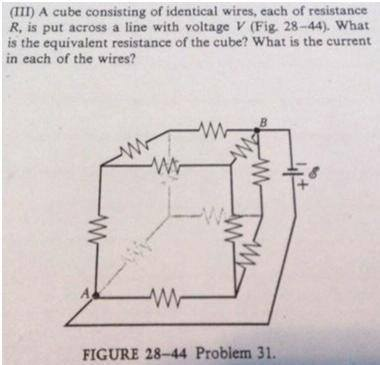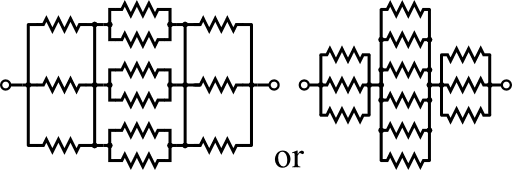# Resistance Cube: Finding current through each resistor## Homework Statement

The problem and picture are attached in an attachment

A cube consisting of identical wires, each of resistance R, is put across a line with voltage V (Fig. 28-44). What is the equivalent resistance of the cube? What is the current in each of the wires?

V=IR

## The Attempt at a Solution

Try drawing it out as a 2D schematic. Then it's just like any other similar problem.

ehild
Homework Helper
There is a trick for solving such symmetrical arrangements of resistors. Find points at equal potential: They can be connected with a wire, it does not change anything, but makes the solution easy.

ehild

Ehild and QuarkChamber are exactly right!

If you connect points of equal potential, you end up with an equivalent current of this form:(image taken from here: resistance cube solution)

I guess you can solve the resistance cube now on your own :)

The network is not reducible to a simple series and parallel combinations of resistors,There is,however a clear symmetry in the problem which we can exploit to obtain the equivalent resistance of the network.
Now the job is to find the sides in which the current is the same,say I.Further,at the corners the incoming current I must split equally into the outgoing branches.In this manner,the current in all the 12 edges of the cube are easily written down in terms of I,using Kirchhoff's first rule and the symmetry in the problem.For example take a closed loop,and apply Kirchhoff's second law $\rightarrow$ -IR-(1/2)IR-IR+ε = 0 ;where R is the resistance of each edge and ε the emf of the battery.
Now find the equivalent resistance R$_{eq}$
For R=R Ω,R$_{eq}$ = ? and for ε=V Volts,the total current in the network is $\longrightarrow$